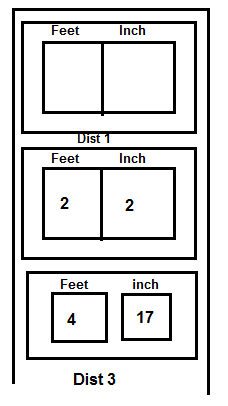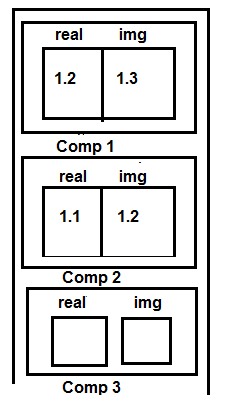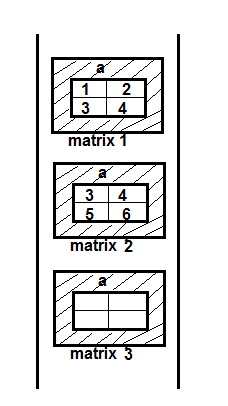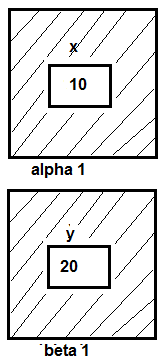Ratings:
(4)
Views:362The mechanism of giving special meanings to an operator is known as operator overloading.

This is one of the exciting features of C++

This technique has enhanced the power of extensibility of C++.

This technique permits to add two user defined variables with the same syntax that is applied to basic types.

Provides the new definitions for most of the C++ operators.

Int x=10;  Matrix M1; Int y=20;  Matrix M2;   all are used under defined data type Int z;        Matrix M3; Z=x+y;    M3=M1+M2;// error

Exisiting operators perform operations only on predefined data types but cannot perform operation on user defined data types

Creating a new definition of an existing operations is called operator overloading.

We can overload all the C++ operators except ,

Class member acess operators(.,.*)

Scope resolution operator(::)

Size of operator(size of)

Conditional operator(?:)

Syntax Return type operator operator –symbol(operand is) { Statements; }

Overloaded operator is a specific function whose name is operator symbol.(int operator+( ) );

Only existing operators can be overloaded. The operator must have at least one operand of user-defined type .New operator cannot be created. We cannot change the basic meaning of the operator.

They cannot be overridden.

When binary operators overloaded through member function, the left hand operand must be an object of the relevant class.

Overload operator is a specific function whose name is operator symbol.

Example # include<iostream.h> Struct distance { float feet; flaot inch; }; distance operator+(distance d1,distance d2) { distance d3; d3.feet=d1.feet+d2.feet; d3.inch=d1.inch+d2.inch; return d3; } void main( ) { distance dist 1,dist 2,dist 3; cout<<”Input distance 1”; cin>>dist1.feet>dist.inch; cout<<”\n Input distance 2”; cin>>dist2.feet>dist2.inch; dist3=dist1+dist2; cout<<”\n Sum is \n”; cout<<dist3.feet<<”\t”<<dist3.inch; }### Overloaded operator as a member function

(11 overload+ operator for adding two complex numbers # include <iostream.h> Class complex { float read; float img; public; void read( ) { Cout<<” In Input read”; Cin>>read; Cout<<” In Input img”; Cin>>img; } void print( ) { Cout<<endl<<real<<”1t”<<img;Complex operator+(complex  c2) { complex C3; C3.real=real+C2.real; C3.img=img+C2.img; return C3; } Void main( ) { Complex comp1,comp2,comp3; Comp1.read( ): Comp2.read( ): Comp3= comp1+comp2; Comp1.print( ); Comp2.print( ); Comp3.print( ); }# include<iostream.h> # include<string.h> class string { char str; public; void read_string( ) { cout<<”Input any string”; cin>>str; } Void print_string( ) { } Int operator==(string S2) { If(strmcmp(str,s2.str)==0) return 1; else return 0; }}; void main ( ) { string str1,str2; Str1.read_string( ); Str2.read_string( ); Str1.print_string( ); Str2.print_string( ); If(str 1==str2) cout<<”In strings are equal”; else cout<<”In strings are not equal”; }

Example #include <iostream.h> class matrix { int a; public: void read_matrix( ) { cout<<”In Input elements of Matrix”; for (int i=0; i<2;i++) for(int j=0; J<2;j++) cin>>a[i][j]; } void print_matrix( ) { for (int i=0;i<2;i++) for ( int j=0;j<2;i++) cout<<a[i][j]<<”lt”; cout<<endl; }} Matriox operator+(matrix M2) { Matirx M3; for ( int i=0;i<2;i++) for ( int j=0;j<2;i++) M3.a[i][j]=a[i][j]+M2.a[j]; return M3; } void Main() { Matrix Matrix1, Matrix2, Matrix3, Matrix1.read_matrix(); Matrix2.read_matrix(); Matrix3=Matrix1+Matrix2; Matrix1.print_Matrix( ); Matrix2.print_Matrix( ); Matrix3.print_Matrix( ); }

A binary operator takes two operandas member function overloading binary operator will have one explicit augument. EX:overloading binary+operator Test operator+(test obj); If test is name of the class and obj1,obj2 and obj3 are the objects of the test then the expression. Obj3=obj1+obj2; Invokes the function overloading binary+operator Obj1 invokes the function and obj2 will be passed as an argument. The function returns the sum of obj1 and obj2 and is assigned to obj3.

Member function overloading unary operators does no have expenditure have explicit arguments. Eg// overloading ++,-- operators --overlaoded unary ++,-- does not diff prefix and postfix both are same.    Example # include <iostream.h> # include<conio.h> Class integer { Int n; Public; Integer(int x) { n=x; } Void operator ++( ){ N=n+5; } Void operator—( ) { n=n-5; } Cout<<endl<<n; }}; Void main( ) { Integer a(10); a.print( ); a++; a.print(); a--; a.print(); }

• If overloaded operator is member, it operates on similar data types/objects
• Friend operator can operate on more than one type of object/data type.
• The operators cannot be overloaded using friend function.

Assignment operator    = Function call operator ( ) Subscripting operator [ } Class member access operator----> Member functions can be used to overload them.

Takes two explicit arguments Ex:friend test operator+(test obj1,test obj2) The function adds the values of the data members of the objects obj1 and obj2 when invoked and return their sum. The syntax for invoking a friend function will be same as that of member function i.e obj3=obj1+obj2; The objects are passed explicitly to the function and their sum is assingned to obj3. Class Alpha                         class beta {                                           { intx;                                      inty; Public:                                   public; Void read_x()                        void read_y() {                                               { Cin>>x;                                    cin>>y; }                                                  } };                                                 };   Example # include<iostream.h> class beta; class alpha { intx; public: void read_x( ) { cout<<”In Input the value of X’; cin>>x; } { Cout<<”In x=”<<x; } Friend int operator==(alpha,beta); }; Class beta { Int y; Public; Void read _y() { Cout<<”In put Y”; Cin>>y; } {void print_Y() { Cout<<’In y=<<y; } Friend int operator ==(alpha,beta); }; Int operator==(alpha a,beta b) { If (a.x==b.y) return 1; else Return 0; } void main ( ) { alpha alpha1; beta beta1; alpha1.read_x(); beta1.read_y(); If (alpha1==beta 1) cout<<”/n no.of objects are equals”; else cout<< “/n no.of objects are not equals”; }### (>>,<<) overloading extraction (..) and insertion(<<) operator for operating on userdefined data types.

Eg:# include<iostream.h> class student { char name; char course; public; friend void operator>> (istream&,student &); friend void operator <<(ostream&,student &); }; void operator>>(istream & in,student & s) in>>s.name; in>>s.course; } void operator <<(ostream & out,student &) { out<<s.name<<endl; out<<s.course; } Void main( ) { Student stud1; Cout>>”/n inter student details”; Cin>>stud1; cout<</n student details are In” }